Metal Displacement Reactions Chemistry Tutorial

Key Concepts

• A more active (reactive) metal will displace a less active (reactive) metal from solution.1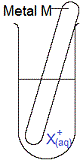A piece of metal M(s) is placed in an aqueous solution of metal X ions, X+(aq).

(i) If metal M is a more active metal than metal X, then metal M displaces metal X from the solution.
The piece of metal M disintegrates as metal M ions, M+(aq), are produced.
Metal X is deposited as solid metallic X, X(s)
Metal M has been oxidised:

M(s) → M+(aq) + e-

Metal M is a stronger reductant (or a stronger reducing agent) than metal X
So the ions of metal X, X+(aq) are reduced to solid X(s):

X+(aq) + e- → X(s)

The more active metal is the metal which is the stronger reductant (that is, the more active metal is easier to oxidise).

(ii) If metal M is less active than metal X, then metal M is the weaker reductant and NO displacement reaction occurs.
Metal M does NOT disintegrate.
Metal X does NOT deposit.

• There are two ways to determine which metal is the more active (reactive):
(i) An activity series can be used because it lists metals in order from most active to least active.

(ii) A table of standard reduction potentials (Eo values) can be used because it lists metals in order from strongest reductant (strongest reducing agent) to weakest reductant (reducing agent).

No ads = no money for us = no free stuff for you!

Metal Displacement Reactions and Activity Series

An activity series can be used to determine which metal is the most active (reactive).
An activity series places metals in order from most active (reactive) to least active (reactive):

 metals most active metals → → → → → → → → → → → least active metals K > Ba > Ca > Na > Mg > Al > Mn > Zn > Fe > Ni > Sn > Pb > Cu > Ag

In this series the most active metal is potassium (K) and the least active metal is silver (Ag).

 Consider an experiment in which you place a clean strip of zinc metal, Zn(s), in an aqueous solution of copper(II) ions, Cu2+(aq) as shown in the diagram on the right: From the activity series above we see that zinc (Zn) is a more active metal than copper (Cu). A more active metal will displace a less active metal from solution. Zinc will displace copper(II) ions from the solution.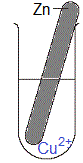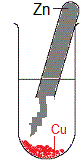Over time, we will observe: (i) The amount of solid zinc will decrease, we say the piece of zinc disintegrates. (ii) Solid copper will deposit, usually seen on the bottom of the test tube.

 Consider another experiment in which you place a clean strip of zinc metal, Zn(s), in an aqueous solution of magnesium ions, Mg2+(aq) as shown in the diagram on the right: From the activity series above we see that zinc (Zn) is a less active metal than magnesium (Mg). A more active metal will displace a less active metal from solution. Magnesium would displace zinc ions from the solution. BUT we do not have a piece of magnesium, and our solution does not contain zinc ions! So, over time, we do not see any changes to the amount of zinc metal present, nor do we see any solid metal being deposited in the test tube.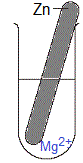Do you know this?

Play the game now!

Metal Displacement Reactions and Strength of Reductant (Reducing Agent)

If you are given an activity series, then it is easy to use that to determine whether a reaction will occur when a piece of metal is placed in an aqueous solution containing the ions of a different metal, just like we did in the preceding examples.

However, usually you will not have an activity series provided, but you will have a table of standard reduction potentials (Eo values).
You can use the table of standard reduction potentials (Eo) to determine whether a reaction will occur when a piece of metal is placed in an aqueous solution containing ions of a different metal.

• A more active (reactive) metal is one that is easier to oxidise:

Metal, M(s), readily loses an electron:
M(s) → M+ + e-

• A more active (reactive) metal is therefore a stronger reductant (reducing agent)
A reductant (or reducing agent) is a species that causes another species to undergo reduction.
The reductant (or reducing agent) is itself oxidised.
• A metal displacement reaction is therefore an example of a redox reaction in which:
(i) One metal is oxidised because it is the stronger reductant (reducing agent)

M(s) → M+(aq) + e-

(ii) The metal in solution is the weaker reductant (reducing agent), so these ions, X-(aq), will be reduced to X(s):

X+(aq) + e- → X(s)

(iii) The overall redox reaction can be represented as:

M(s) + X+(aq) → M+(aq) + X(s)

Solid metal M is oxidised to ions, M+(aq), which become part of the aqueous solution.
The electrons released are used to reduce the ions of metal X in solution, X+(aq), to produce solid metallic X, X(s).
We say that metal M has displaced metal X from the solution.
• A table of standard reduction potentials (Eo values) lists substances in order from strongest reductant to weakest reductant:

E0(volts)

Weakest
Oxidant
K+ + e- K(s) -2.94 Strongest
Reductant
Ba2+ + 2e- Ba(s) -2.91
Ca2+ + 2e- Ca(s) -2.87
Na+ + e- Na(s) -2.71
Mg2+ + 2e- Mg(s) -2.36
Al3+ + 3e- Al(s) -1.68
Mn2+ + 2e- Mn(s) -1.18
Zn2+ + 2e- Zn(s) -0.76
Fe2+ + 2e- Fe(s) -0.44
Ni2+ + 2e- Ni(s) -0.24
Sn2+ + 2e- Sn(s) -0.14
Pb2+ + 2e- Pb(s) -0.13
Cu2+ + 2e- Cu(s) 0.34
Strongest
Oxidant
Ag+ + e- Ag(s) 0.80 Weakest
Reductant

In the table above, the strongest reductant (reducing agent) is potassium, K(s), while the weakest reductant (reducing agent) is silver, Ag(s).

 Consider an experiment in which you place a clean piece of iron, Fe(s), in an aqueous solution containing silver ions, Ag+(aq), as shown in the diagram on the right. Using the table of standard reduction potentials above, we see that iron, Fe(s), is a stronger reductant than silver, Ag(s). That is, metallic iron is easier to oxidise than metallic silver. In other words, solid iron loses electrons more easily than solid silver. Therefore we expect: (i) iron will be oxidised to iron ions Fe(s) → Fe2+(aq) + 2e- (ii) silver ions will be reduced to solid metallic silver Ag+(aq) + e- → Ag(s) That is, we expect iron to displace silver from the solution because iron is a stronger reductant than silver.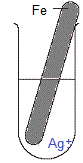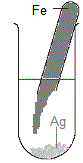Over time, we expect to observe: (i) The piece of solid iron disintegrating. (ii) Solid silver being deposited.

We can use the equations for the oxidation and reduction reactions above to write an overall redox reaction equation to represent the displacement reaction:

Oxidation: Fe(s) → Fe2+(aq) + 2e-

Note: 2 electrons are produced for each atom of iron that is oxidised.

Both electrons must be used in the reduction of silver ions to silver metal:

Reduction: Ag+(aq) + e- → Ag(s)
Only 1 electron is used to reduce a silver ion to a silver atom
Therefore we need to multiply each species in the equation by 2 (so that we can use 2 electrons in the equation)
2Ag+(aq) + 2e- → 2Ag(s)

Now we add the oxidation equation to the reduction equation:

Oxidation: Reduction: Overall: Fe(s) → 2e- + Fe2+(aq) 2e- + 2Ag+(aq) → 2Ag(s) Fe(s) + 2Ag+(aq) → 2Ag(s) + Fe2+(aq)

 Now consider a different experiment in which you place a clean piece of iron, Fe(s), in an aqueous solution containing sodium ions, Na+(aq), as shown in the diagram on the right. Using the table of standard reduction potentials above, we see that sodium, Na(s), is a stronger reductant than iron, Fe(s). That is, metallic sodium is easier to oxidise than metallic iron. In other words, solid sodium loses electrons more easily than solid iron. Therefore we expect: (i) sodium ions, Na+(aq), will NOT be reduced to solid sodium, Na(s), because if it were, the solid sodium would oxidise back to sodium ions! Na(s) → Na+(aq) + e- (ii) iron atoms, Fe(s), will NOT be oxidised to iron ions, if it were, the iron ions would immediately be reduced back to iron atoms: Fe2+(aq) + 2e- → Fe(s) That is, we DO NOT expect iron to displace sodium from the solution because iron is a weaker reductant than sodium.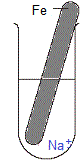Do you understand this?

Take the test now!

Worked Example

 Question: Chris the Chemist pours blue aqueous copper sulfate solution, CuSO4(aq), into a test tube until it is half full. After thoroughly cleaning a strip of magnesium metal until it is shiny, Chris cuts off a piece that is about the same length as the test tube and places it in the test tube as shown in the diagram on the right. Chris observes the experiment for 10 minutes. What observations do you expect to find in Chris's lab notes?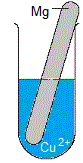Solution:

(Based on the StoPGoPS approach to problem solving.)

1. What is the question asking you to do?

Predict what will be observed as this experiment proceeds.

2. What data (information) have you been given in the question?

Extract the data from the question:
Solid metal is magnesium, Mg(s)
Solution is blue aqueous copper(II) sulfate, CuSO4(aq)
Table of Standard Reduction Potentials (data sheet)

3. What is the relationship between what you know and what you need to find out?

(i) When copper(II) sulfate dissolves in water to form an aqueous solution, copper(II) ions (Cu2+(aq)) and sulfate ions (SO42-(aq)) will be present in the solution.

(ii) If magnesium metal is a stronger reductant than copper metal, then:

(a) magnesium atoms will be oxidised to magnesium ions
Mg(s) → Mg2+(aq) + 2e-

(b) and copper ions will be reduced to copper atoms
Cu2+(aq) + 2e- → Cu(s)

(iii) If magnesium metal is a weaker reductant than copper metal, no displacement reaction will occur.

(iv) Refer to the Table of Standard Reduction Potentials.
Magnesium is a stronger reductant than copper so magnesium atoms will be oxidised and copper ions will be reduced.

(i) The piece of magnesium metal disintegrates, it is visibly smaller in size as the experiment proceeds.
(magnesium ions are being formed and these enter the solution)

(ii) Solid copper deposits in the test-tube.
Solid copper will probably look red-gold or red-brown in colour.
Because the solid copper is more dense than water, it will usually fall to the bottom of the test tube.

(iii) The blue colour of the copper(II) sulfate solution will fade, that is, over time the solution will be less blue in colour.
As the copper(II) ions are consumed in the production of copper atoms, the concentration of copper(II) ions decreases which results in a decrease in the intensity of the blue colour in the test tube.

Use some logic based on your understanding of the relative reactivity of magnesium and copper metals.

Magnesium is a Group 2 metal that you should know is quite reactive.
A metal is reactive if it loses electrons easily, that is, if it can be easily oxidised.
A metal that is easily oxidised is a strong reductant.
One of the reasons you do not see household objects made of magnesium is because it is reactive, BUT, it is used in things like fire-starters because it readily reacts.

Copper, on the other hand, is not a very reactive (active) metal.
People in ancient times used copper to make household goods because the metal can be found in its native (unreacted) state. Even today you will still find copper being used to make household items.
Clearly then, copper metal is not so easily oxidised, that is, it is not as active as magnesium, and therefore copper is a weaker reductant than magnesium.

Given the opportunity, magnesium atoms will oxidise to magnesium ions, and copper ions will reduce to copper atoms.

So, our observations about the mass of magnesium atoms decreasing, the concentration of copper ions decreasing, and the deposition of solid copper, is looking plausible.

6. State your solution to the problem:

Observations:
(i) The piece of magnesium becomes smaller in size over time.
(ii) A new red-brown solid is seen in the test tube.
(iii) The blue colour of the solution fades over time.

Can you apply this?

Join AUS-e-TUTE!

Take the exam now!

1. We are going to ignore any other competing reactions during this discussion.
Note that water is an even weaker reductant than silver metal.
½O2(g) + 2H+(aq) + 2e- ⇋ H2O(l)   Eo = +1.23 V